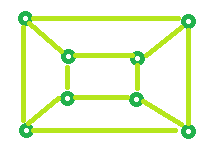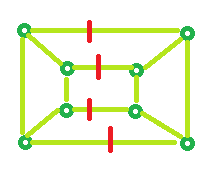# Program to Calculate the Edge Cover of a Graph

Given the number of vertices N of a graph. The task is to determine the Edge cover.

Edge Cover: Minimum number of edge required to cover all vertex is known as Edge Cover.

Examples:

```Input : N = 5
Output : 3

Input : N = 4
Output : 2
```

## Recommended: Please try your approach on {IDE} first, before moving on to the solution.

Example 1: For N = 5 vertices,Edge Cover is: 3 (Choosing the edges marked in Red, all of the vertices will get covered)Example 2: For N = 8 vertices,Edge Cover is: 4 (Choosing the edges marked in Red, all of the vertices will get covered)Formula:

```Edge Cover = ceil (no. of vertices / 2)
```

Below is the implementation of the above approach:

## C++

 `// C++ program to find Edge Cover ` `#include ` `using` `namespace` `std; ` ` `  `// Function that calculates Edge Cover ` `int` `edgeCover(``int` `n) ` `{ ` `    ``float` `result = 0; ` ` `  `    ``result = ``ceil``(n / 2.0); ` ` `  `    ``return` `result; ` `} ` ` `  `// Driver Code ` `int` `main() ` `{ ` `    ``int` `n = 5; ` ` `  `    ``cout << edgeCover(n); ` ` `  `    ``return` `0; ` `} `

## Java

 `// Java program to find Edge Cover ` `import` `java.util.*; ` `import` `java.lang.*; ` `import` `java.io.*; ` ` `  `class` `GFG{ ` `// Function that calculates Edge Cover ` `static` `int` `edgeCover(``int` `n) ` `{ ` `    ``int` `result = ``0``; ` `  `  `    ``result = (``int``)Math.ceil((``double``)n / ``2.0``); ` `  `  `    ``return` `result; ` `} ` `  `  `// Driver Code ` `public` `static` `void` `main(String args[]) ` `{ ` `    ``int` `n = ``5``; ` `  `  `    ``System.out.print(edgeCover(n)); ` `} ` ` `  `} `

## Python3

 `# Python 3 implementation of the above approach. ` ` `  `import` `math ` ` `  `# Function that calculates Edge Cover  ` `def` `edgeCover(n): ` `     `  `    ``result ``=` `0` `     `  `    ``result ``=` `math.ceil(n ``/` `2.0``) ` `     `  `    ``return` `result ` `     `  `     `  `# Driver code       ` `if` `__name__ ``=``=` `"__main__"` `:  ` `   `  `    ``n ``=`  `5` `   `  `    ``print``(``int``(edgeCover(n))) ` ` `  `# this code is contributed by Naman_Garg `

## C#

 `// C# program to find Edge Cover ` `using` `System; ` ` `  `class` `GFG ` `{ ` `// Function that calculates Edge Cover ` `static` `int` `edgeCover(``int` `n) ` `{ ` `    ``int` `result = 0; ` ` `  `    ``result = (``int``)Math.Ceiling((``double``)n / 2.0); ` ` `  `    ``return` `result; ` `} ` ` `  `// Driver Code ` `static` `public` `void` `Main () ` `{ ` `    ``int` `n = 5; ` `     `  `    ``Console.Write(edgeCover(n)); ` `} ` `} ` ` `  `// This code is contributed by Raj `

## PHP

 ` `

Output:

```3
```

Don’t stop now and take your learning to the next level. Learn all the important concepts of Data Structures and Algorithms with the help of the most trusted course: DSA Self Paced. Become industry ready at a student-friendly price.

My Personal Notes arrow_drop_upCheck out this Author's contributed articles.

If you like GeeksforGeeks and would like to contribute, you can also write an article using contribute.geeksforgeeks.org or mail your article to contribute@geeksforgeeks.org. See your article appearing on the GeeksforGeeks main page and help other Geeks.

Please Improve this article if you find anything incorrect by clicking on the "Improve Article" button below.

Article Tags :
Practice Tags :

Be the First to upvote.

Please write to us at contribute@geeksforgeeks.org to report any issue with the above content.